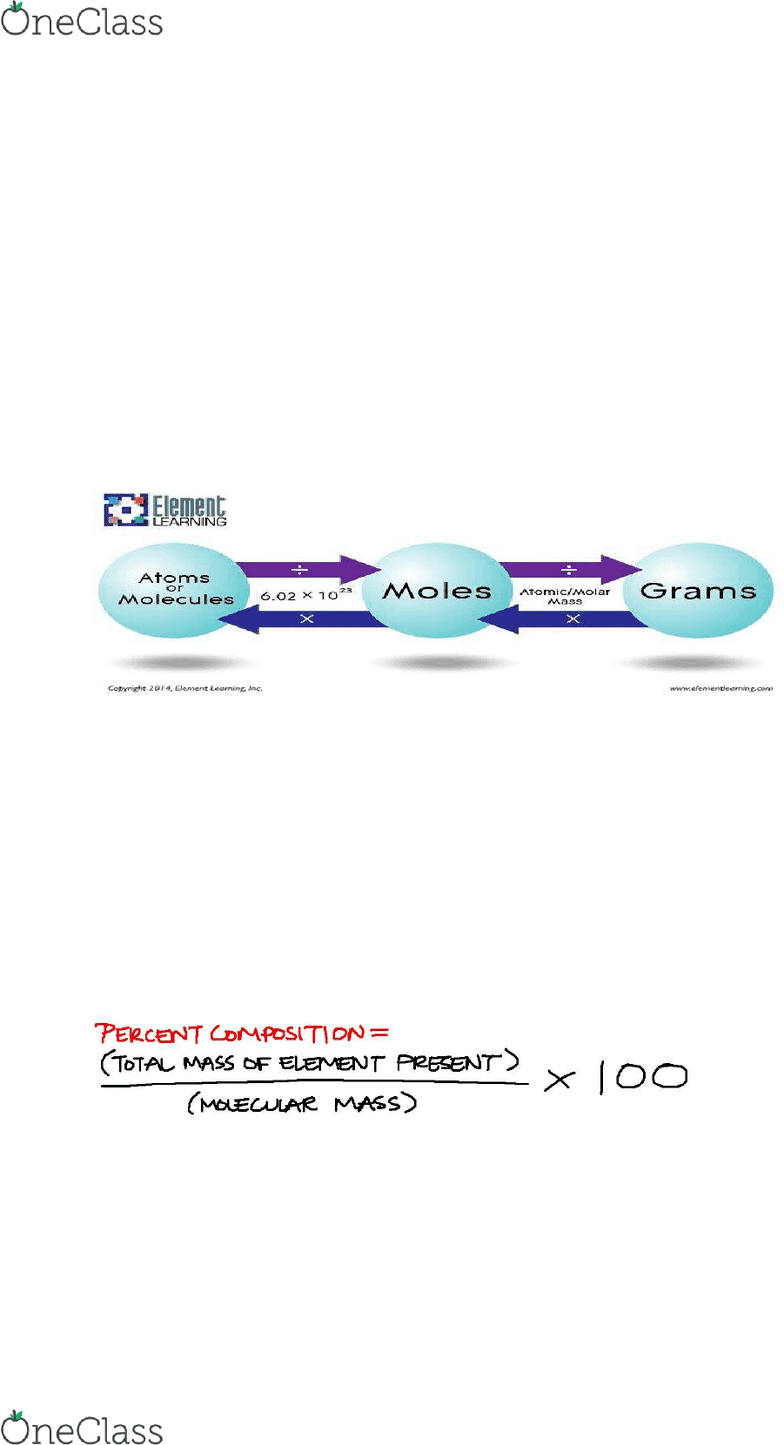Textbook Notes (280,000)
US (110,000)
CHM (9)
Chapter 3

# CHM 111 Chapter Notes - Chapter 3: Mass Spectrometry, Limiting Reagent, Unified Atomic Mass Unit

This preview shows half of the first page. to view the full 3 pages of the document.CHAPTER 3
Atomic mass: The mass of an atom in atomic mass units (amu)
1amu = 1/12 the mass of one carbon-12 atom
o 12amu = mass of one carbon-12 atom
Average atomic mass: weighted measurement of mass of elements, accounts for naturally
occurring isotopes & their varying abundance
o Calculated using mass spectrometer, multiply % abundance by atomic mass, take sum
of all isotopic forms
Mole (mol): SI unit for the amount of substance that contains as many elementary entries
(atoms, molecules, etc.) as there are atoms in exactly 12g of carbon-12 isotope
o 1mol = # atoms in 12g of carbon-12
Avogadro's #: NA = 6.0221413 E 23
Molar mass: mass of 1 mole of units of a substance () (g/mol) (kg/mol)
o eg. 12g of carbon-12 atoms / NA carbon-12 atoms = 1.993 E -23 g
Mass of 1 carbon-12 atom
Molecular mass: sum of all the atomic masses in a molecule (in amu)
o eg. H2O : 2(atomic mass of H) + (atomic mass of O) = molecular mass
o **Molar mass of compound (g) = molecular mass (amu)**
Ionic compounds - formula mass: sum atomic masses of each ion
o Since electrons have a nominal mass, the elemental atomic mass can be used
Mass spectrometer: gaseous atoms are bombarded by electrons, creating cations that are
reflected; magnitude of these reflections indicates the mass of each ion → discovery of rare
isotopes & average atomic mass
Converting % mass to empirical formula
1. Take mass %, convert to grams
2. Divide mass in grams by molar mass to get moles of each element
3. Take moles of each element, divide by smallest # of moles to get mole ratios
4. Convert mole ratios to integer subscripts to get the empirical formula
Experimental determination of empirical formulas/molecular formulas
find more resources at oneclass.com
find more resources at oneclass.com